PHY_10_38_V2_LP_Capac.energy
Оценка 4.9

# PHY_10_38_V2_LP_Capac.energy

Оценка 4.9
docx
07.05.2020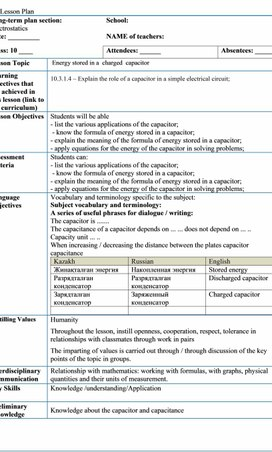PHY_10_38_V2_LP_Capac.energy.docx

Lesson Plan

Long-term plan section:  Electrostatics

School:

Date: _________

NAME of teachers:

Class: 10 ____

Attendees: ______

Absentees: _____

Lesson Topic

Energy stored in a  charged  capacitor

Learning objectives that are achieved in this lesson (link to the curriculum)

10.3.1.4  Explain the role of a capacitor in a simple electrical circuit;

Lesson Objectives

Students will be able

- list the various applications of the capacitor;

- know the formula of energy stored in a capacitor;

- explain the meaning of the formula of energy stored in a capacitor;

- apply equations for the energy of the capacitor in solving problems;

Assessment  Criteria

Students can:

- list the various applications of the capacitor;

- know the formula of energy stored in a capacitor;

- explain the meaning of the formula of energy stored in a capacitor;

- apply equations for the energy of the capacitor in solving problems;

Language Objectives

Vocabulary and terminology specific to the subject:

Subject vocabulary and terminology:

A series of useful phrases for dialogue / writing:

The capacitor is .......

The capacitance of a capacitor depends on ... ... does not depend on ... ..

Capacity unit ... ..

When increasing / decreasing the distance between the plates capacitor capacitance

 Kazakh Russian English Жинақталған энергия Накопленная энергия Stored energy Разрядталған конденсатор Разрядталған конденсатор Discharged capacitor Зарядталған конденсатор Заряженный конденсатор Charged capacitor

Instilling Values

Humanity

Throughout the lesson, instill openness, cooperation, respect, tolerance in relationships with classmates through work in pairs

The imparting of values is carried out through / through discussion of the key points of the topic in groups.

Interdisciplinary Communication

Relationship with mathematics: working with formulas, with graphs, physical quantities and their units of measurement.

Key Skills

Knowledge /understanding/Application

Preliminary Knowledge

Knowledge about the capacitor and capacitance

Lesson

Scheduled Lesson Stages

Scheduled Lesson Activities

Resources

Beginning

8 min

At the beginning of the lesson, teacher introduces the students to the topic of the lesson, discusses with students LO of the lesson.

Checking h/w and if necessary some of problems can be solved on the board.

Starter:

Teacher ask questions and pick students who will answer, rest can help and suggest their versions of answers.

Review questions:

-          What is a capacitor?

-          What are the main parts of it?

-          Define capacitance?

-          What is the formula for the parallel plate capacitor capacitance?

-          Name the applications of the capacitors?

Slides

Middle

10 min

10 min

Activity 2:

http://electricalschool.info/spravochnik/eltehustr/1509-dlja-chego-v-jelektricheskikh-cepjakh.html

Students work in small groups and read the text and make notes in a form of table and title it.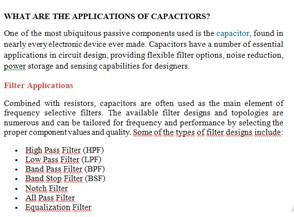The teacher looks through the students' notebooks, as they are completed. At the end each group present 1 application of capacitors.

Activity 3: Energy stored in a capacitor

Students watch a video and working in a small group or in pairs answer to the questions: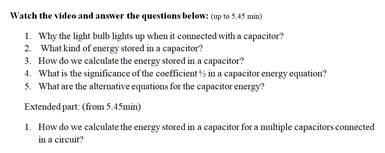laptops if available

10 min

Speaker from each group present the answer to the 1 question and teacher might complete his answer if it is necessary.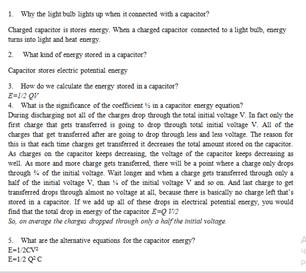Teacher ask questions each group and might complete students answers in order to make sure that everything is clear to them.

When all questions answered teacher gives a worksheet and students start to solve problems on a new topic.

1.    A parallel-plate air capacitor has a capacitance of 100 pF with a charge of magnitude 0.1 µC on each plate. The plates are 0.5 mm apart.

2.    (a) What is the potential difference between the plates?

(b) What is the area of each plate?

(c) What is the electric-field magnitude between the plates?

9. A parallel plate capacitor consists of two 5.0 cm x 5.0 cm metal electrodes spaced 1.5 mm apart. The capacitor is connected to a 10 V battery. How much energy is stored in the capacitor?

If students couldn’t finish solve these problems they continue it at home.

Worksheets, calculators

Students write on sticker if they fully understand the topic, or if they write partially teacher ask them specify the question.

Homework: repeat on the textbook, the material studied, and solve the problem

5.(a) Name the two applications of capacitors in an electrical circuit.

1...................................................................................

2.............................................................................. 

For example, smoothing DC ripple, energy storage, blocking P.T. generator circuits, tuning circuits, synchronization circuits.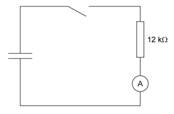(b) The figure shows a circuit containing a capacitor, a resistor of 12 kΩ, a switch and a sensitive ammeter. The capacitor is charged to a potential difference of 15 V.

After the key is closed, the current in the circuit changes over time.

Describe the relationship between the current in the circuit and the charge passing through a point in the circuit.

………………………………………………………………… 

(i)                 Answer: the charge is equal to the strength of the current multiplied by the time of its passage.

At the initial time, the charge on the capacitor was 3.3 x 103 μC, with a potential difference of 15V. Calculate the capacitance of the capacitor.

C = ................................................... μF 

worksheets

End

2 min

Reflection: Have students answer the following questions on post it notes or small pieces of paper.

-       What have you learned?

-       What remains unclear?

What do you still need to work on?

Differentiation - how do you plan to provide more support? What tasks do you plan to set for more capable students?

Grading - how do you plan to check students' level of learning?

Health & Safety

Support for students is planned to be provided through discussion of the created model of the reference scheme while working with the text of the article, then through checking the basic formulas by the teacher and advising while solving problems.

Through observing by individual students solving problems during the lesson

Creating a favorable psychological atmosphere, the compliance of tasks to the level of preparedness of students, the change of activities, counseling.

What tasks do you plan to set for more capable students?

Providing advice to students who have difficulty

Students will:

Most students will:

Some learners will be:

Students can:

- list the various applications of the capacitor;

- know the formula of energy stored in a capacitor;

- explain the meaning of the formula of energy stored in a capacitor;

- apply equations for the energy of the capacitor in solving problems;

- explain how to find the capacitor energy when multiple capacitors connected in series or parallel

Lesson reflection

Use this section to reflect on the lesson. Answer the most important questions about your lesson from the left column.

Were the objectives of the lesson / learning objectives realistic?

Have all students reached the DH?

If not, why not?

Is the differentiation correct in the lesson?

Have the lesson temporal stages been sustained?

What were the deviations from the lesson plan and why?

What are two aspects of the lesson that went well (think about both teaching and learning)?

1:

2:

What could contribute to improving the lesson (think about both teaching and learning)?

1:

2:

What did I discover during the lesson about the class or the achievements / difficulties of individual students, what should I look for in the subsequent lessons?

Скачано с www.znanio.ru

## Teacher ask questions each group and might complete students answers in order to make sure that everything is clear to them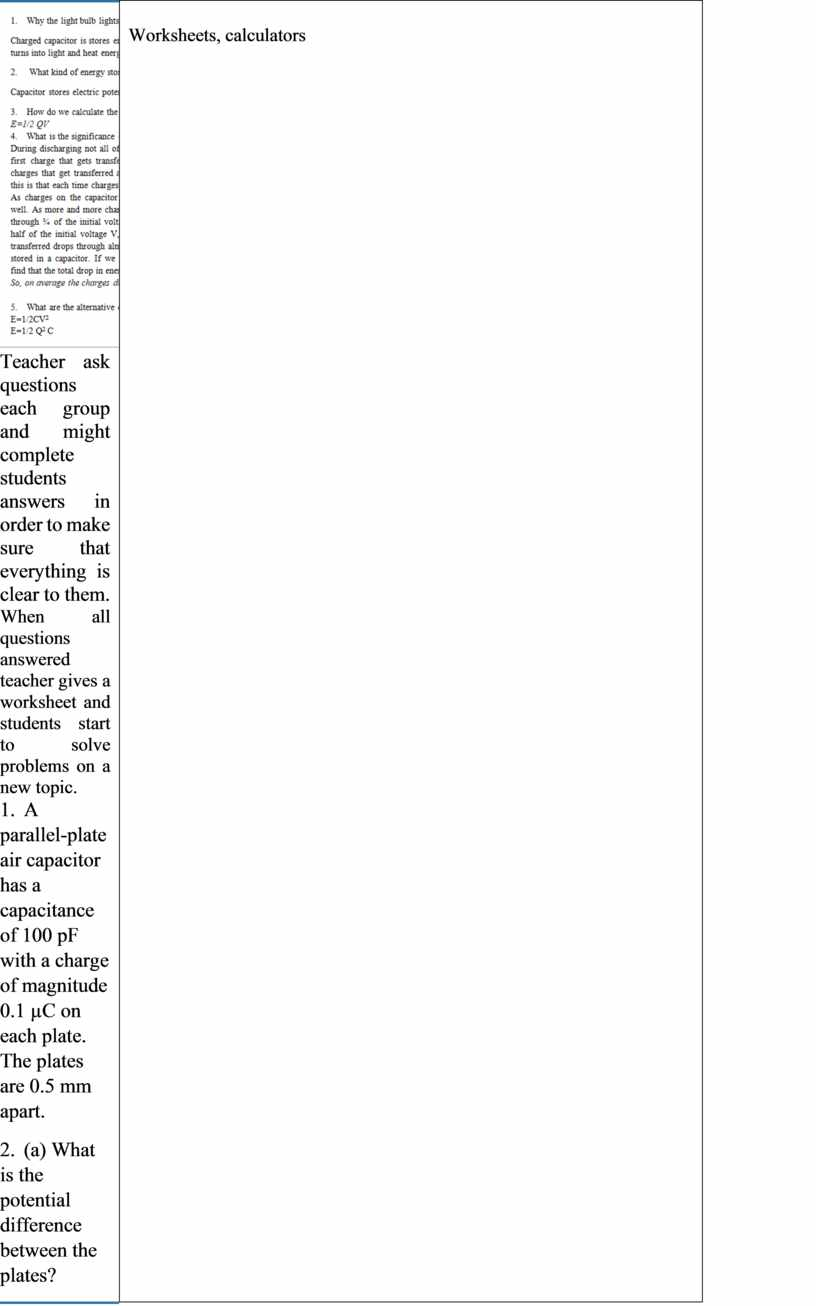## What did I discover during the lesson about the class or the achievements / difficulties of individual students, what should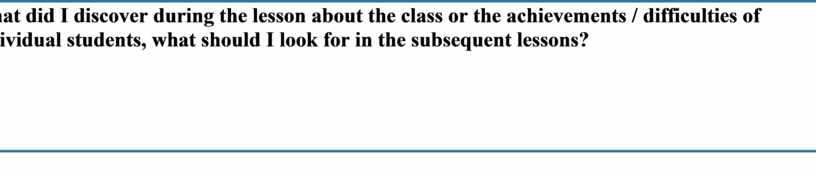Скачать файл